# Texas Go Math Kindergarten Lesson 12.1 Answer Key Subtraction as Separating

Refer to our Texas Go Math Kindergarten Answer Key Pdf to score good marks in the exams. Test yourself by practicing the problems from Texas Go Math Kindergarten Lesson 12.1 Answer Key Subtraction as Separating.

## Texas Go Math Kindergarten Lesson 12.1 Answer Key Subtraction as Separating

Explore

DIRECTIONS: Listen to the subtraction word problem. Use cubes. Trace the number that shows how many children in all. Trace the minus symbol and the number that shows how many children are leaving. Write the is equal to symbol and the number that shows how many children are left.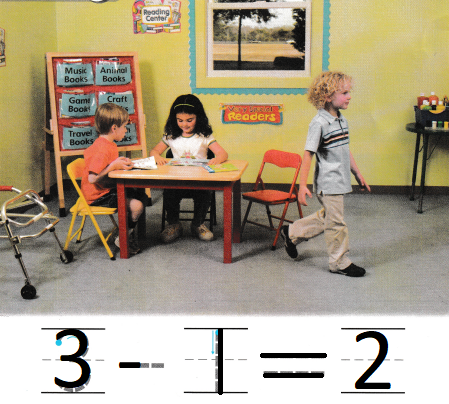Explanation:
There are 3 children in the class room
one child is leaving
so, 3 – 1 = 2
2 children are remaining

Share and Show

DIRECTIONS: 1. Listen to the subtraction word problem. Use cubes. Write the number that shows how many children in all. Trace the minus symbol and write the number that shows how many children leave. Trace the is equal to symbol and write the number that shows how many children are still at the table.

Question 1.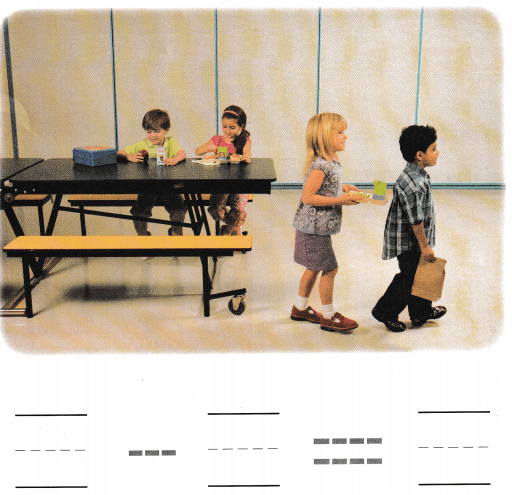Explanation:
There are 4 children in the class room eating their lunch
2 completed the food and leaving
so, 4 – 2 = 2
2 children are still eating their food.

DIRECTIONS: 2. Listen to the subtraction word problem. Use cubes. Write the number that shows how many children in all. Trace the minus symbol and write the number that shows how many children leave. Trace the is equal to symbol and write the number that shows how many children are still in the block center.

Question 2.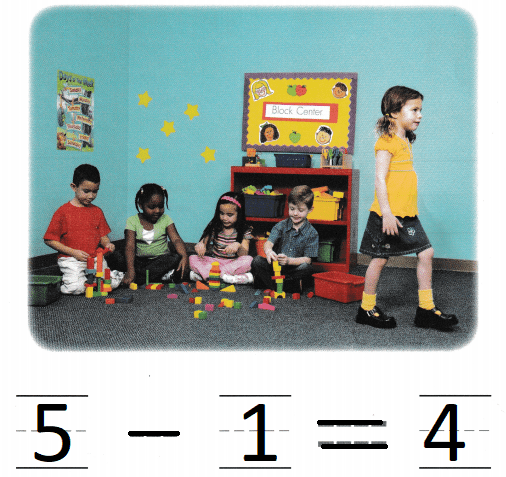Explanation:
There are 5 children in the class room playing with blocks
one child is leaving
so,5 – 1 = 4
2 children are still playing with the blocks.

HOME ACTIVITY • Show your child a set of four small objects. Have him or her tell how many objects there are. Take one of the objects from the set. Have your child tell you how many objects there are now.

DIRECTIONS: 3. Blair has two marbles. He gives one marble to his friend. How many marbles does Blair have now? Draw to show the subtraction. Write the numbers and trace the symbols to complete the subtraction sentence. 4. Choose the correct answer. Use cubes. Five children went to the park together. Then two children leave. How many children are staying at the park?

Problem Solving

Question 3.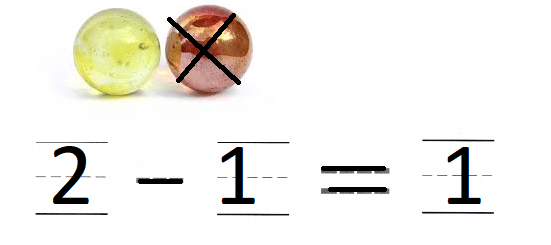Explanation:
Blair has two marbles. He gives one marble to his friend.
2 – 1 = 1
Blair have now 1 marble.

Question 4.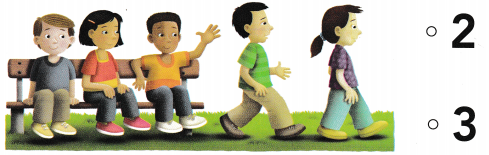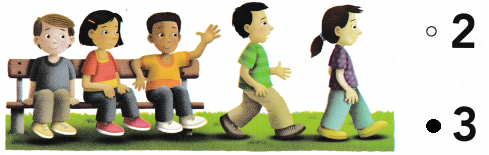Explanation:
Five children went to the park together.
Then two children leave
5 – 2 = 3
so, 3 children are remaining.

### Texas Go Math Kindergarten Lesson 12.1 Homework and Practice Answer Key

DIRECTIONS: 1. Four children ore waiting in line. Then one child leaves. Write the number that shows how many children in all. Write the number that shows how many children are leaving. Write the number that shows how many children are still in line.

Question 1.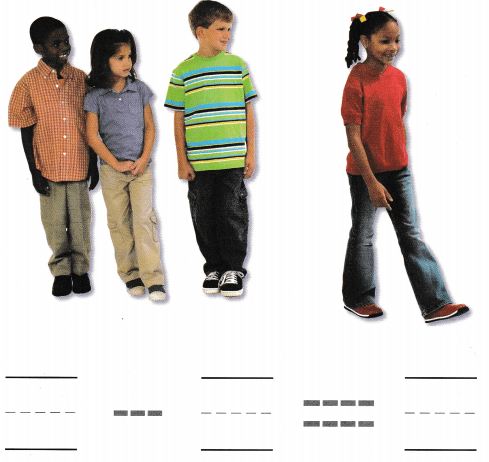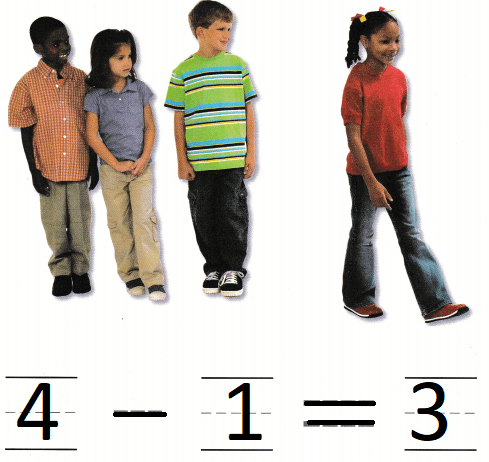Explanation:
Four children ore waiting in line. Then one child leaves.
4 – 1 = 3
so, 3 children are remaining.

DIRECTIONS: Choose the correct answer. 2. Three birds are sitting. Then two birds fly away. How many birds are left? 3. Five horses are eating. Then three horses run away. How many horses are left?

Lesson Check

Question 2.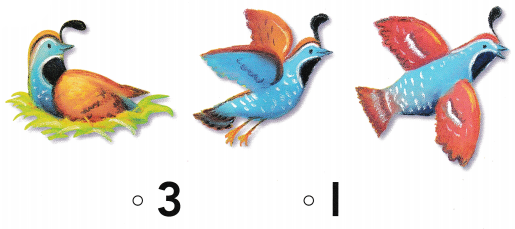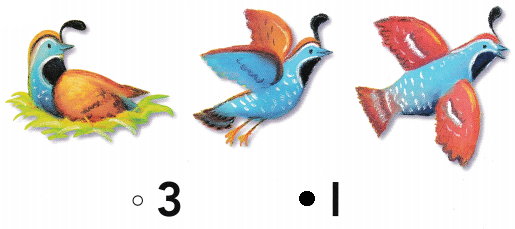Explanation:
Three birds are sitting. Then two birds fly away.
3 – 2 = 1
so, 1  bird is left

Question 3.